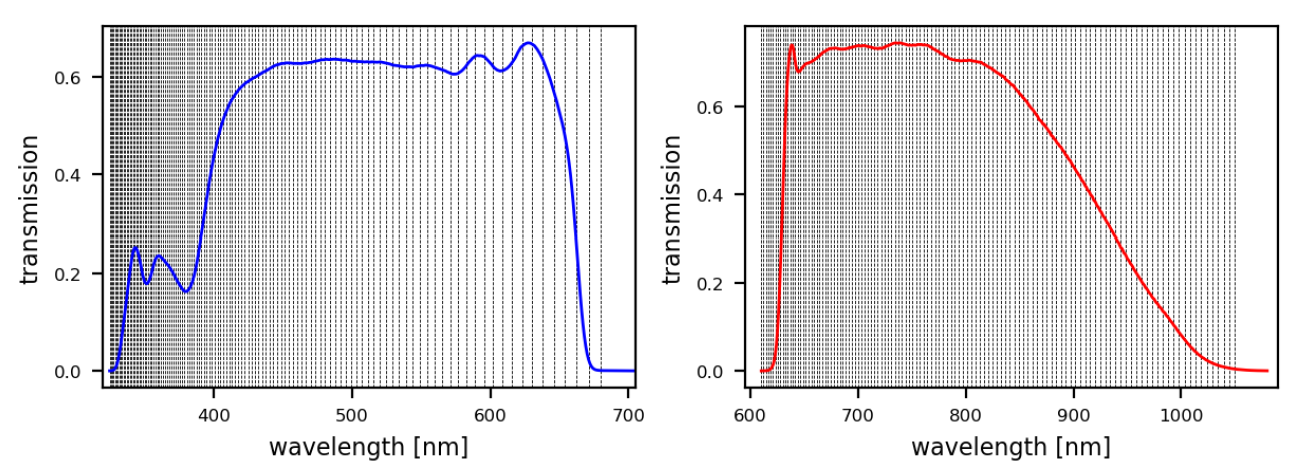# 11.3.1 Sampled Mean Spectrum generator (SMSgen)

Author(s): René Andrae

## Goals

SMSgen is a purely technical module, running first in the Apsis chain. Its tasks comprise the following: First and foremost, SMSgen provides sampled low-resolution BP/RP spectra as input to the Apsis modules. More specifically, SMSgen provides the integrated fluxes and the flux uncertainties in 120 pixels per BP and RP spectrum. Second, SMSgen applies the correction to the flux in the $G$ band as proposed by Riello et al. (2021), see Section 11.2.2. Third, SMSgen applies the zero-point correction to the parallax, see Section 11.2.1.

## Inputs

SMSgen uses the continuous representation of internally calibrated BP/RP spectra in terms of coefficients and a table of pre-defined physical wavelengths (converted to pixel position with the applicable dispersion relation from CU5). All 55 coefficients for both, BP and RP, are used, i.e. SMSgen does not use the truncation methods proposed in Carrasco et al. (2021).

## Method

Given the basis definition for the continuous representation of internally calibrated BP/RP spectra chosen by CU5, SMSgen computes a “design matrix” $D$ which provides the integrated flux of each basis function in all 120 pixels. These pixels cover the wavelength ranges for non-zero transmission in BP and RP, as is shown in Figure 11.5. For a given coefficient vector $\boldsymbol{c}$, the sampled BP/RP spectrum $\boldsymbol{s}$ is then given by the simple matrix product $\boldsymbol{s}=D\cdot\boldsymbol{c}$. This is done for BP and RP coefficients separately, providing sampled spectra for BP and RP, respectively, each having 120 pixels. The flux uncertainties are propagated from the coefficient covariance matrix $\Sigma_{\textrm{coeffs}}$ provided by CU5. SMSgen computes the full pixel covariance matrix $\Sigma_{\textrm{spec}}=D\cdot\Sigma_{\textrm{coeffs}}\cdot D^{T}$. However, the full pixel covariance matrices for BP and RP each have format $120\times 120$ and thus consume a lot of memory. Since in DR3 no Apsis module actually accounts for correlated errors in pixel fluxes, SMSgen only provides the standard deviations for each pixel, i.e. the square-roots of the diagonal elements in $\Sigma_{\textrm{spec}}$. Besides, with only 55 coefficients provided by CU5 for each BP and RP, $\Sigma_{\textrm{spec}}$ cannot have full rank and thus could not be inverted for usage in a $\chi^{2}$.Figure 11.5: Wavelengths of SMSgen sampling for BP (left panel) and RP (right panel). The solid blue and red lines show the DR3 transmission curves for BP and RP, respectively. Each panel shows the 121 edges of the 120 pixels as vertical dashed black lines. The pixels are approximately equidistant in pixel space, but due to the nonlinear dispersion their width is varying in wavelength space.

## Scope

SMSgen does not provide any outputs in DR3. It produced sampled BP/RP spectra for all sources for internal usage only by Apsis processing.